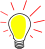## 6.2. gpioserver, basic GPIO-commands

The basic GPIO layer command list is:

• Inputs: `config` + pin number + ( `in` | `input` )
• Inputs with pullup: `input` + pin number + ( `inpullup` | `inputpullup` )
• Inputs with pulldown: `input` + pin number + ( `inpulldown` | `inputpulldown` )
• Inputs with no pull-whatever: `input` + pin number + ( `inpullnone` )
• Outputs: `config` + pin number + ( `out` | `output` )
• Output PWM: `config` + pin number + ( `outputpwm` )

to set a gpio, use `gpio` + pin number + ( `on` | `high` | `off` | `low` )

For example, `gpio17on`.

to set a pwm rate, use `gpio` + pin number + pwm + [ 0..1024 ] (according to my experiments, 0..100 is the range)Whatever configuration is set, it is possible to send a GPIO-command and an input will react as an output. Think this is more a bug than a feature.